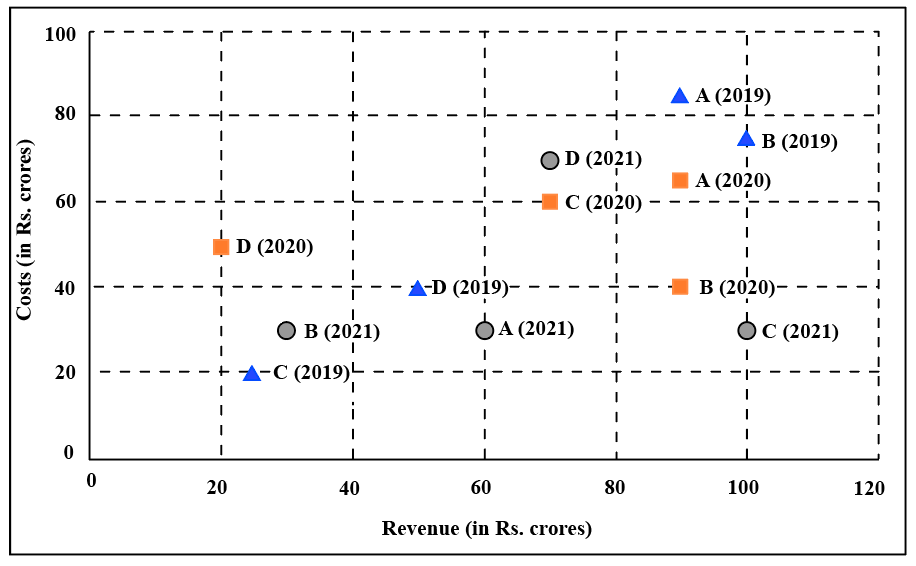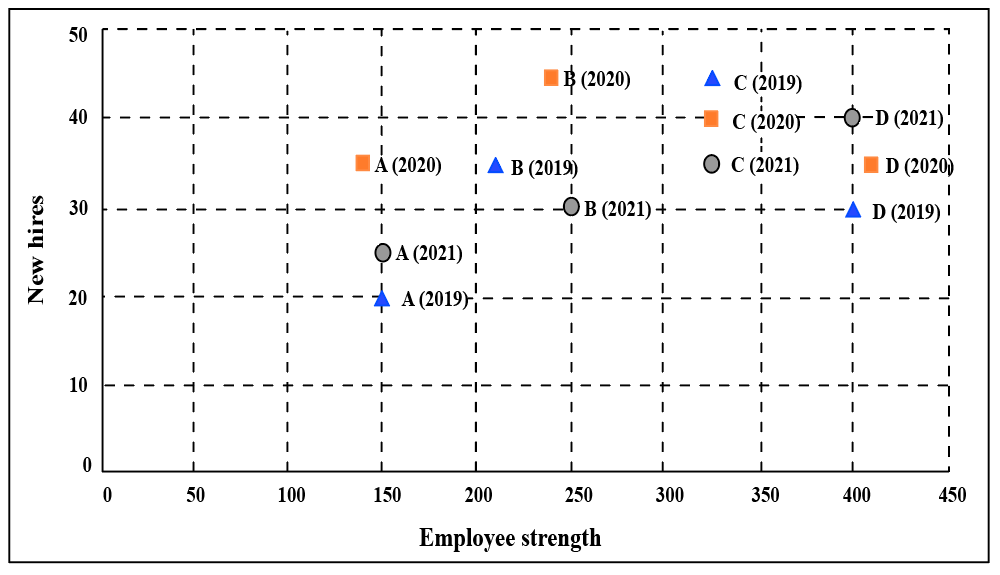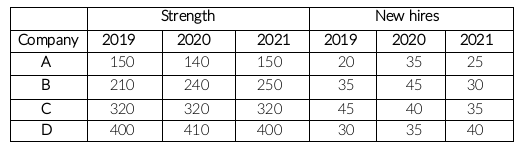### CAT 2022 Question Paper (Slot 2) Question 38

Instructions

The two plots below show data for four companies code-named A, B, C, and D over three years - 2019, 2020, and 2021.

The first plot shows the revenues and costs incurred by the companies during these years. For example, in 2021, company C earned Rs.100 crores in revenue and spent Rs.30 crores. The profit of a company is defined as its revenue minus its costsThe second plot shows the number of employees employed by the company (employee strength) at the start of each of these three years, as well as the number of new employees hired each year (new hires). For example, Company B had 250 employees at the start of 2021, and 30 new employees joined the company during the year.Question 38

# The total number of employees lost in 2019 and 2020 was the least for:

Solution

It is given,Company A:

The number of employees in the beginning of 2019 = 150

The number of employees hired in 2019 = 20

The number of employees should be at the beginning of 2020 is 150+20, i.e. 170 but there are 140 only. This implies 30 left company A in 2019.

The number of employees in the beginning of 2020 = 140

The number of employees hired in 2020 = 35

The number of employees should be at the beginning of 2021 is 140+35, i.e. 175 but there are 150 only. This implies 25 left company A in 2020.

The number of employees left company A in 2019 and 2020 = 30 + 25 = 55

Company B:

Similarly, the number of employees left company B in 2019 = 210 + 35 - 240 = 5

The number of employees left company B in 2020 = 240 + 45 - 250 = 35

The number of employees left company B in 2019 and 2020 = 5 + 35 = 40

Company C:

Similarly, the number of employees left company C in 2019 = 320 + 45 - 320 = 45

The number of employees left company C in 2020 = 320 + 40 - 320 = 40

The number of employees left company C in 2019 and 2020 = 45 + 40 = 85

Company D:

Similarly, the number of employees left company D in 2019 = 400 + 30 - 410 = 20

The number of employees left company D in 2020 = 410 + 35 - 400 = 45

The number of employees left company D in 2019 and 2020 = 20 + 45 = 65

The total number of employees lost in 2019 and 2020 is least for company B.